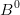## Constraint fromThe mixing induced CP asymmetry in thedecay allows one to determine the sine of the anglealmost without any hadronic uncertainties. In this case the CP asymmetry appears in the interference between amplitudes describing decays with and without mixing. The simplest case is when the final state is a specific CP state. The following time dependent asymmetry is studied:The decay is dominated by a single (tree level) amplitude (the same process can be described by a Penguin diagram which brings corrections at order). It implies thatand the expression becomes:The constraint onis obtained combining the experimental measurement of thedecay to the data-driven determination of the theoretical error, obtained from the time-dependent asymmetry ofand the determination of decay rate and the C parameter of the time-dependent asymmetry in, following the prescription of hep-ph/0507290. The constraint onfromdecays toand higher charmonium states is not included, since the experimental information to determine the theoretical error on that is missing.

The experimental values we use are summarized in the Table of Inputs. The representation of this constraint in theplane is given below.EPS - PDF - PNG - JPG - GIF

Ideas, requests, problems regarding this web site? Send feedback